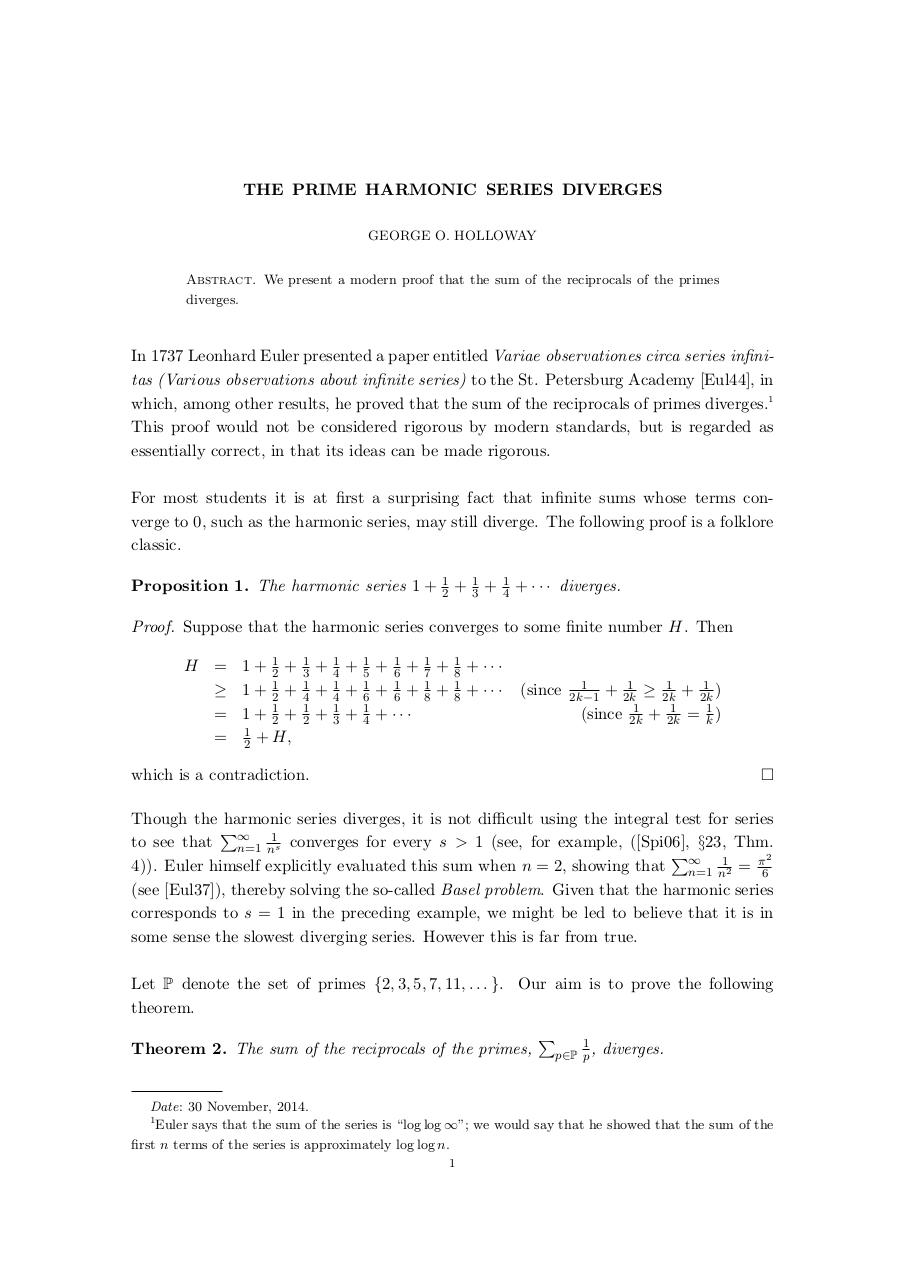# PDF Archive

Easily share your PDF documents with your contacts, on the Web and Social Networks.

## EulerPrimeRecips.pdfPage 1 2 3

#### Text preview

THE PRIME HARMONIC SERIES DIVERGES
GEORGE O. HOLLOWAY
Abstract. We present a modern proof that the sum of the reciprocals of the primes
diverges.

In 1737 Leonhard Euler presented a paper entitled Variae observationes circa series infinitas (Various observations about infinite series) to the St. Petersburg Academy [Eul44], in
which, among other results, he proved that the sum of the reciprocals of primes diverges.1
This proof would not be considered rigorous by modern standards, but is regarded as
essentially correct, in that its ideas can be made rigorous.
For most students it is at first a surprising fact that infinite sums whose terms converge to 0, such as the harmonic series, may still diverge. The following proof is a folklore
classic.
Proposition 1. The harmonic series 1 +

1
2

+

1
3

+

1
4

+ · · · diverges.

Proof. Suppose that the harmonic series converges to some finite number H. Then
H = 1 + 21 +
≥ 1 + 12 +
= 1 + 12 +
= 12 + H,

1
3
1
4
1
2

+
+
+

1
4
1
4
1
3

+
+
+

1
5
1
6
1
4

+ 16 +
+ 16 +
+ ···

1
7
1
8

+
+

1
8
1
8

+ ···
+ ···

(since

1
2k−1

1
1
1
+ 2k
≥ 2k
+ 2k
)
1
1
1
(since 2k + 2k = k )

Though the harmonic series diverges, it is not difficult using the integral test for series
P
1
to see that ∞
n=1 ns converges for every s &gt; 1 (see, for example, ([Spi06], §23, Thm.
P
1
π2
4)). Euler himself explicitly evaluated this sum when n = 2, showing that ∞
n=1 n2 = 6
(see [Eul37]), thereby solving the so-called Basel problem. Given that the harmonic series
corresponds to s = 1 in the preceding example, we might be led to believe that it is in
some sense the slowest diverging series. However this is far from true.
Let P denote the set of primes {2, 3, 5, 7, 11, . . . }. Our aim is to prove the following
theorem.
Theorem 2. The sum of the reciprocals of the primes,

P

1
p∈P p ,

diverges.

Date: 30 November, 2014.
1
Euler says that the sum of the series is “log log ∞”; we would say that he showed that the sum of the
first n terms of the series is approximately log log n.
1